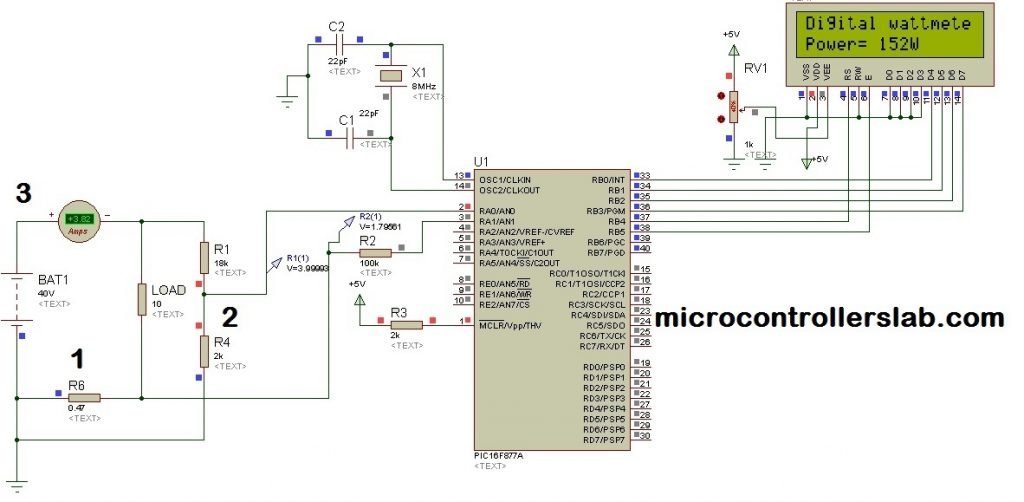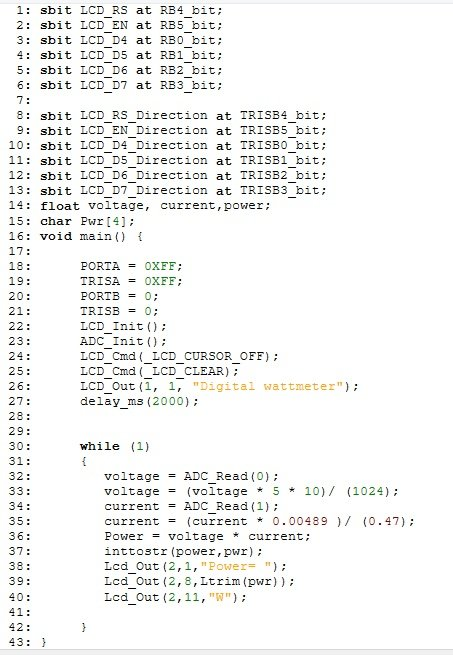# Digital DC watt meter using pic microcontroller

### Digital DC watt meter project using pic microcontroller

Digital dc watt meter using pic microcontroller is used to measure dc power of dc circuits. Voltage and current sensors are used in this project. Voltage and current sensors are interfaced with microcontroller. Voltage sensor is used to measure voltage across circuit or load. Current sensor is used to measure current passing through load. Voltage divider circuit is used to measure high voltage. because microcontroller can not read high voltage or voltage more than 5 volt. Shunt resistor is used as a current sensor.Shunt resistor is used to convert current into voltage form. Because microcontroller does not understand current. Microcontroller can read voltage directly. Liquid crystal display is used to show measured value of dc power. Digital dc watt meter is very simple to use and basic idea of digital dc watt meter is given in this project.

### Digital dc watt meter main components

To understand this project completely you should know how to measure dc voltage using pic microcontroller? How to measure dc current using pic microcontroller? How to interface LCD with pic microcontrollers? If you don’t know about it, I recommend you to read following articles first. So that you can get better understanding of digital dc watt meter project.

I hope that you have gone through above three article and understand them completely. If yes, you can easily design digital dc watt meter using instructions given below.

### Digital dc watt meter circuit diagram

circuit diagram of digital dc watt meter is given below. 0.47 resistor is used as a shunt resistor and I have explained its purpose in digital ammeter project in detail. Resistor R1 and R4 is used as a voltage divider. I have explained their functions in digital voltmeter project. LCD displays measured DC power by fetching its value from PIC16F877A microcontroller. 8MHz crystal is used to operate PIC16F877A microcontroller. DC ammeter used in below circuit is just for simulation purpose. you don’t need to connect it while designing dc watt meter circuit.### Digital Dc watt meter working

As I have mentioned above this project consists of three main components.  Detail of these three components is given below:

• Digital Ammeter : Digital Ammeter is designed using shunt resistor. R6 0.47 shunt resistor is used to measure current flowing through a load. Shunt resistor converts current passing through it into voltage. This voltage is measured with the help of analog channel AN1 of PIC16F877A microcontroller. Measured voltage converted back into current using ohm’s law formula I = V/R. Because value of shunt resistor and measured voltage is known.
• Digital voltmeter: Digital voltmeter is used to measure voltage across load. voltage divider is used to step down voltage less than 5 volt. Voltage across R4 resistor of voltage divider is measured with the help of analog channel  AN0 of PIC16F877A microcontroller. Measured voltage converted back into actual voltage by multiplying it with opposite of voltage divider formula.
• PIC16F877A microcontroller: All mathematical calculations are done through programming of PIC16F877A microcontroller. It reads current and voltage through analog to digital converter. As you know dc power is just a product of voltage and current.

DC Power = Voltage × current

• So by using above formula dc power can be easily calculated by writing simple multiplication instruction in program. I will discuss it later in programming part.

### Video lecture on dc power measurement

Watch this video lecture on Dc power measurement using pic microcontroller, if you want complete step by step guide

:

### Digital dc watt meter code

Code for dc measurement project is written using Mikro pro C compiler. Its program is given below:• voltage = ADC_Read(0) :  This function reads analog value of voltage and converts it into binary value of voltage.
• voltage = (voltage * 5 * 10)/ (1024) : It converts binary value back into actual voltage by multiplying it with ADC resolution factor and voltage divider inverse. You can get its more detail from voltage measurement article.
• current = (current * 0.00489 )/ (0.47) : This function converts binary value of  voltage across shunt resistor into current.
• Power = voltage * current : It’s just a multiplication factor of measured voltage and measured current.
• inttostr(power,pwr) : It converts power value into string.

you may also like to read following article:

### 9 thoughts on “Digital DC watt meter using pic microcontroller”

1. voltage = (voltage * 5 * 10)/ (1024)???
What is 10 here??
Explain sir.

• Multiplication factor for Voltage divider

2. how will convert 40 volt dc to 220 volt dc????

3. How connect the wattmeter for open circuit test of transformer ?the meter have 1to 10 slot for insert the wires and nothing have any other terminal .send mi the connection diagram on this wattsapp no 7507536671

4. I have implement to 60V and 100A what should i implement to this circuit?